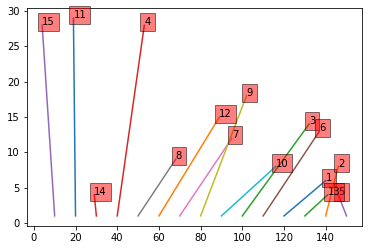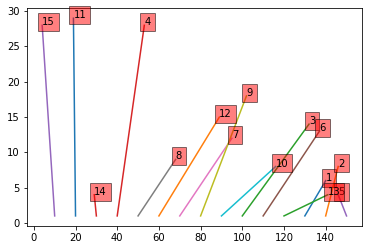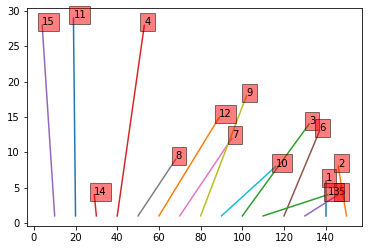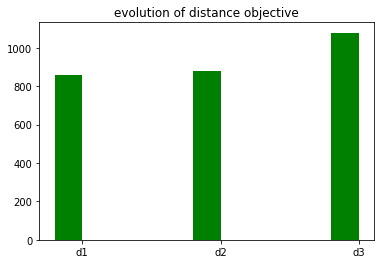# Objects in boxes¶

This tutorial includes everything you need to set up IBM Decision Optimization CPLEX Modeling for Python (DOcplex), build a Mathematical Programming model, and get its solution by solving the model on the cloud with IBM ILOG CPLEX Optimizer.

When you finish this tutorial, you’ll have a foundational knowledge of Prescriptive Analytics.

This notebook is part of Prescriptive Analytics for Python

It requires either an installation of CPLEX Optimizers or it can be run on IBM Watson Studio Cloud (Sign up for a free IBM Cloud account and you can start using Watson Studio Cloud right away).

• We wish to put $$N$$ objects which are scattered in the plane, into a row of $$N$$ boxes.
• Boxes are aligned from left to right (if $$i < i'$$, box $$i$$ is to the left of box $$i'$$) on the $$x$$ axis.
• Box $$i$$ is located at a point $$B_i$$ of the $$(x,y)$$ plane and object $$j$$ is located at $$O_j$$.
• We want to find an arrangement of objects such that:
• each box contains exactly one object,
• each object is stored in one box,
• the total distance from object $$j$$ to its storage box is minimal.
• First, we solve the problem described, and then we add two new constraints and examine how the cost (and solution) changes.
• From the first solution, we impose that object #1 is assigned to the box immediately to the left of object #2.
• Then we impose that object #5 is assigned to a box next to the box of object #6.

## How decision optimization can help¶

• Prescriptive analytics (decision optimization) technology recommends actions that are based on desired outcomes. It takes into account specific scenarios, resources, and knowledge of past and current events. With this insight, your organization can make better decisions and have greater control of business outcomes.

• Prescriptive analytics is the next step on the path to insight-based actions. It creates value through synergy with predictive analytics, which analyzes data to predict future outcomes.

• Prescriptive analytics takes that insight to the next level by suggesting the optimal way to handle that future situation. Organizations that can act fast in dynamic conditions and make superior decisions in uncertain environments gain a strong competitive advantage.

With prescriptive analytics, you can:

• Automate the complex decisions and trade-offs to better manage your limited resources.
• Take advantage of a future opportunity or mitigate a future risk.
• Proactively update recommendations based on changing events.
• Meet operational goals, increase customer loyalty, prevent threats and fraud, and optimize business processes.

## Use decision optimization¶

### Step 1: Import the library¶

Run the following code to import the Decision Optimization CPLEX Modeling library. The DOcplex library contains the two modeling packages, Mathematical Programming and Constraint Programming, referred to earlier.

If CPLEX is not installed, install CPLEX Community edition.

### Step 2: Model the data¶

The input data is the number of objects (and boxes) N, and their positions in the (x,y) plane.

### Step 3: Prepare the data¶

We use Euclidean distance to compute the distance between an object and its assigned box.

### Step 4: Set up the prescriptive model¶

* system is: Windows 64bit
* Python version 3.7.3, located at: c:\local\python373\python.exe
* docplex is present, version is (2, 11, 0)
* pandas is present, version is 0.25.1


#### Create the DOcplex model¶

The model contains all the business constraints and defines the objective.

#### Define the decision variables¶

• For each box $$i$$ ($$i$$ in $$1..N$$) and object $$j$$ ($$j$$ in $$1..N$$), we define a binary variable $$X_{i,j}$$ equal to $$1$$ if and only if object $$j$$ is stored in box $$i$$.

• The sum of $$X_{i,j}$$ over both rows and columns must be equal to $$1$$, resulting in $$2\times N$$ constraints.
Model: boxes
- number of variables: 225
- binary=225, integer=0, continuous=0
- number of constraints: 30
- linear=30
- parameters: defaults
- problem type is: MILP


#### Express the objective¶

• The objective is to minimize the total distance between each object and its storage box.

#### Solve the model¶

Model: boxes
- number of variables: 225
- binary=225, integer=0, continuous=0
- number of constraints: 30
- linear=30
- parameters: defaults
- problem type is: MILP

* model boxes solved with objective = 8858
* solution: [15, 11, 14, 4, 8, 12, 7, 9, 10, 3, 6, 1, 13, 2, 5]


As an additional constraint, we want to impose that object #1 is stored immediately to the left of object #2. As a consequence, object #2 cannot be stored in box #1, so we add:

docplex.mp.LinearConstraint[](x_1_2,EQ,0)


Now, we must state that for $$k \geq 2$$ if $$x[k,2] == 1$$ then $$x[k-1,1] == 1$$; this is a logical implication that we express by a relational operator:

Model: boxes
- number of variables: 225
- binary=225, integer=0, continuous=0
- number of constraints: 45
- linear=45
- parameters: defaults
- problem type is: MILP


Now let’s solve again and check that our new constraint is satisfied, that is, object #1 is immediately left to object #2

* model boxes solved with objective = 8878
solution #2 =[15, 11, 14, 4, 8, 12, 7, 9, 10, 3, 6, 13, 1, 2, 5]


The constraint is indeed satisfied, with a higher objective, as expected.

Now, we want to add a second constraint to state that object #5 is stored in a box that is next to the box of object #6, either to the left or right.

In other words, when $$x[k,6]$$ is equal to $$1$$, then one of $$x[k-1,5]$$ and $$x[k+1,5]$$ is equal to $$1$$; this is again a logical implication, with an OR in the right side.

We have to handle the case of extremities with care.

* model boxes solved with objective = 9078
solution #3 =[15, 11, 14, 4, 8, 12, 7, 9, 10, 3, 13, 6, 5, 1, 2]


As expected, the constraint is satisfied; objects #5 and #6 are next to each other. Predictably, the objective is higher.

### Step 5: Investigate the solution and then run an example analysis¶

Present the solution as a vector of object indices, sorted by box indices. We use maptplotlib to display the assignment of objects to boxes.

The first solution shows no segments crossing, which is to be expected.The second solution, by enforcing that object #1 must be to the left of object #2, introduces crossings.OrderedDict([('d1', 858), ('d2', 878), ('d3', 1078)])## Summary¶

You learned how to set up and use IBM Decision Optimization CPLEX Modeling for Python to formulate a Mathematical Programming model and solve it with CPLEX.